This is part 2 of the blog on GeoPandas, in which we will complete the example workflow. The steps remaining now are to generate some random points around Victoria (to simulate addresses), create some rotated bounding boxes for our postcodes, and join the postcodes to our random points.

### Worked Example, Pt. 2

Let’s continue.

Geopandas Example (Part 2)
In :
import geopandas


## Create some random points¶

To simulate the point-based locations, let's generate some random points within Victoria.

Let's import the dissolved Victoria shape to create these points.

In :
vic_shape = geopandas.read_file('./outputs/vic_shape.shp')
ax = vic_shape.plot()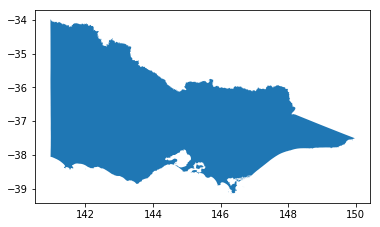We can then use the convex_hull property to get a simplified shape for processing

In :
vic_hull = vic_shape.convex_hull
ax = vic_hull.plot()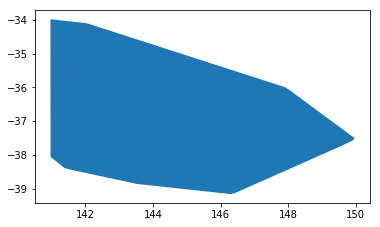To generate some points, we need to be able to create the points and assign them random coordinates.

For the points, we can use shapely geometry's Point() constructor; and for the randomness, numpy's random.random() function - so let's import both of those

In :
import numpy as np
from shapely.geometry import Point


I'm going to generate the points using the max bounds of the convex hull, which we can access using the bounds property. This returns a DataFrame object, with bounds values stored in its columns.

(there is only one feature in the convex hull GeoDataFrame, so we can access the first member directly with 

In :
xmin, xmax, ymin, ymax = vic_hull.bounds['minx'], \
vic_hull.bounds['maxx'], \
vic_hull.bounds['miny'], \
vic_hull.bounds['maxy']

Out:
minx miny maxx maxy
0 140.961682 -39.15919 149.976679 -33.980426

random.random(2000) returns an iterable series of 2000 random numbers, which we then multiply by the difference between the maxs and mins, and add to the min.

We can then use the python builtin zip() function and some list comprehension to generate a series of 2000 random cooridnate pairs, which we can then put into a GeoDataFrame.

In :
xc = (xmax - xmin) * np.random.random(2000) + xmin
yc = (ymax - ymin) * np.random.random(2000) + ymin

points_geom = geopandas.GeoSeries([Point(x, y) for x, y in zip(xc, yc)])

points = geopandas.GeoDataFrame(geometry=points_geom, crs=vic_shape.crs)


The result:

In :
ax = points.plot()

Out:
geometry
0 POINT (143.960365400963 -35.05311960089848)
1 POINT (142.1390937133225 -35.23316358347386)
2 POINT (145.6092495145762 -34.67539365503112)
3 POINT (143.7396759588953 -37.80429592873785)
4 POINT (146.2956772119603 -34.17576970698816)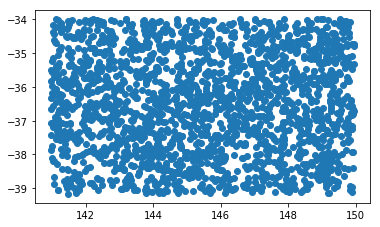## Clipping and Joining¶

Now that we have our random points, I want to both trim them to those that fall within our Victorian postcodes, and assign them a postcode.

So let's get our postcodes data that we saved earlier.

In :
vic_poas = geopandas.read_file('./outputs/vic_poas_plus.shp', crs=vic_shape.crs)
ax = vic_poas.plot()

Out:
POA_NAME code state index_righ geometry
0 5440 5440 None VIC POLYGON ((139.354282080054 -33.15319775377327,...
1 2648 2648 None VIC POLYGON ((141.1528471038108 -34.07935328182845...
2 2717 2717 None VIC POLYGON ((142.028479295783 -34.12086824387433,...
3 2735 2735 None VIC POLYGON ((143.5917940163808 -35.07012300167514...
4 2736 2736 None VIC POLYGON ((143.3328978881271 -35.02792139375725...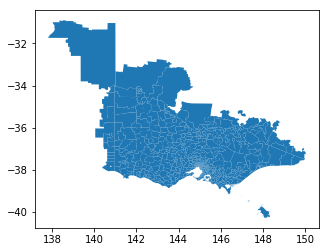Then we can actually do the clip and join in a single step, using once again the GeoPandas excellent spatial join function sjoin(), and the GeoDataFrame query() method.

We can perform the join directly (the overlay method defaults to intersection), and then filter in one line of code using query('index_right >= 0'), such that we only keep records that were successfully joined.

(Note that rows wihtout joins have a value of NaN, so anything with a value >= 0 will clip out any unjoined points)

In :
vic_points = geopandas.sjoin(points, vic_poas, how='left').query('index_right >= 0')
ax = vic_points.plot()

Out:
geometry index_right POA_NAME code state index_righ
1 POINT (142.1390937133225 -35.23316358347386) 112.0 3507 3507.0 VIC VIC
3 POINT (143.7396759588953 -37.80429592873785) 242.0 3352 3352.0 VIC VIC
5 POINT (144.3871868870758 -36.07645350845647) 301.0 3573 3573.0 VIC VIC
7 POINT (143.5243637639433 -34.71312819289769) 153.0 2715 2715.0 None VIC
8 POINT (141.4733579060588 -37.44605650168303) 52.0 3312 3312.0 VIC VIC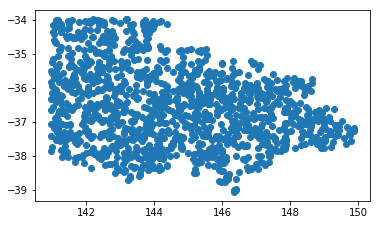Our GeoDataFrame has some extra data that we don't need, so let's trim those columns off using the drop() method.

In :
vic_points = vic_points.drop(['index_right','index_righ','code'], axis=1)

Out:
geometry POA_NAME state
1 POINT (142.1390937133225 -35.23316358347386) 3507 VIC
3 POINT (143.7396759588953 -37.80429592873785) 3352 VIC
5 POINT (144.3871868870758 -36.07645350845647) 3573 VIC
7 POINT (143.5243637639433 -34.71312819289769) 2715 None
8 POINT (141.4733579060588 -37.44605650168303) 3312 VIC

## Creating bounding boxes¶

The last step is to create some bounding boxes for the postcodes. We can do this too in one line of code using the envelope property, then plot it (with some colour customisation this time)

In :
ax = vic_poas.envelope.plot(edgecolor='red', facecolor='none')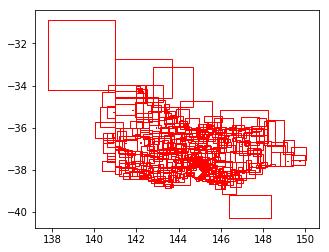However, these bounding boxes are square - I'd rather have them rotated to represent the orientation of underlying polygon better.

For this we can use the shapely property minimum_rotated_rectangle. This property isn't available (yet?) on a GeoDataFrame or GeoSeries object, but the geometries in our GeoDataFrame are stored as shapely geometries, so we can still access them by going to the geometries directly.

In :
print(type(vic_poas['geometry']))
vic_poas['geometry']



Out:
In :
vic_poas['geometry'].minimum_rotated_rectangle

Out:

To get the minimum_rotated_rectangle for each postcode area, we can iterate over the rows in the GeoDataFrame by using the iterrows() method, and using list comprehension, build a GeoSeries with geometries populated from the minimum_rotated_rectangle property.

In :
box_calcs = geopandas.GeoSeries([ item['geometry'].minimum_rotated_rectangle for idx, item in vic_poas.iterrows() ])

boxes = geopandas.GeoDataFrame(geometry=box_calcs)

ax = boxes.plot(edgecolor='red', facecolor='none')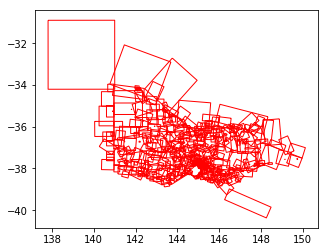Finally, let's rejoin the postcode column to our newly generated boxes. We can just slap the column directly onto the end using boxes['postcode'] = vic_poas['POA_NAME'] because the boxes were generated directly from the same data and won't lose their order

In :
boxes['postcode'] = vic_poas['POA_NAME']

Out:
geometry postcode
0 POLYGON ((137.8099941610417 -30.90466653587301... 5440
1 POLYGON ((143.6931717827698 -32.90458627442858... 2648
2 POLYGON ((141.9662174835874 -34.16551342395636... 2717
3 POLYGON ((143.2779633067582 -35.19909099623962... 2735
4 POLYGON ((143.4667536962667 -34.81306904164063... 2736

Now we have both our rotated bounding boxes and random points with postcodes, let's plot them together:

In :
final_plot = boxes.plot(edgecolor='red', facecolor='none')
vic_points.plot(ax=final_plot, marker='.', color='green')

Out: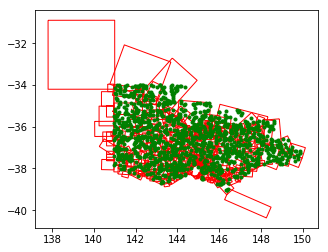And that's it!

Latest posts by Tom Hollands (see all)